# SBI PO Prelims Reasoning Questions 2019 – Day 01

Practice with our SBI PO Prelims reasoning questions 2019 day 1. The Exam pattern for SBI PO has been changing every year. Due to the dynamic changes in exam pattern, the questions become tougher compared to the previous year. So the questions are at a high level than the candidate’s calculation.

Depends upon the current situation our IBPS Guide is providing the updated SBI PO prelims reasoning questions 2019 day 1 for SBI PO 2019. Our Skilled experts were composing the questions under the new level of the examination and in aspirant’s exam point of view. So candidates gear up your preparation, practice daily with our SBI PO Prelims reasoning questions 2019 day 1 and implement your skill. With your attentive preparation, we wish you all getting success in your SBI PO 2019.

“Success usually comes to those who are too busy to be looking for it”

[WpProQuiz 4965]

### Click Here for SBI PO Pre 2019 High-Quality Mocks Exactly on SBI Standard

Directions (1 – 5): Study the following information carefully and answer the given questions.

Eleven boxes – P, Q, R, S, T, U, V, W, X, Y and Z are placed one above other. The boxes were numbered 1 to 11 from bottom to top but not necessarily in the same order.

The box number of Box W is thrice that of box number of Box Y. Box V is placed just below Box Y. There are two boxes between Box Q and Box P. Box P is kept at one of the positions above Box Q. Sum of positions of Boxes U and V was equal to the position of Box P. Box Z is kept just above Box T. Box X is kept at one of the prime numbered positions. Only two boxes are kept between Box S and Box X. Box X is placed at one of the positions above Box S. At least there are four boxes below Box T. Box P is placed at one of the positions above 6.

1) What is the sum of positions of Box X and Box R?

a) 10

b) 9

c) 8

d) 7

e) 6

2) How many boxes are placed above T?

a) More than three

b) Three

c) Two

d) One

e) None

3) How many boxes remain at the same position if the boxes are made to arrange from bottom to top in alphabetical order?

a) One

b) More than four

c) Three

d) Two

e) None

4) Which of the following statement is/are true?

a) Box S is at one of the position above Box T

b) Box Q is placed at even numbered position

c) There are more than two boxes between Box U and S

d) Number of boxes above Box Y is as same as the number of boxes below Box W.

e) None are true

5) Which of the following box is placed at Position 8?

a) Box P

b) Box Q

c) Box S

d) Box R

e) Box V

Directions (6 – 9): Study the following information carefully and answer the given questions.

456 547 623 526 375

6) If ‘1’ is added to each odd digit, ‘2’ is added to each even digit, then all then all the digits are arranged in ascending order within the number, which of the following number is the lowest number?

a) 375

b) 526

c) 456

d) 623

e) None of these

7) If first and third digits are interchanged then 5 is added to even number and 4 is subtracted from odd number, then what is the product of third digit of the highest number with second digit of the second lowest number?

a) 10

b) 6

c) 12

d) 4

e) None of these

8) If 2 is added to the digit which is below 5, 1 is subtracted from the digit which is above 5 (include 5 also), how many digit appear twice within the number?

a) Two

b) Three

c) One

d) None

e) None of these

9) If first and third digits are interchanged, then 2 is added to 1st digit of each number, and 1 is subtracted from second digit of each number, then what is the sum of first digit of the highest number and second digit of the third lowest number?

a) 8

b) 12

c) 14

d) 11

e) None of these

10) Using 1st, 2nd, 4th, 5th, 6th and 9th letter of the word ‘COMPENDIUM’ a meaningful word can be formed. What will be the second last letter of the newly formed word? If more than one word can be formed mark ‘X’ and if no word can be formed mark ‘Y’.

a) C

b) Y

c) E

d) O

e) X

Directions (1 – 5):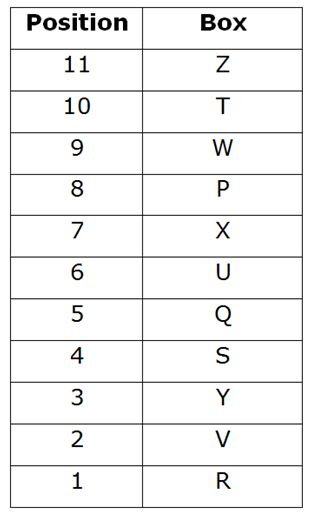• The box number of Box W is thrice that of box number of Box Y.
• Box V is placed just below Box Y.

Since, Box V is placed just below Y. Case-(1) gets eliminated.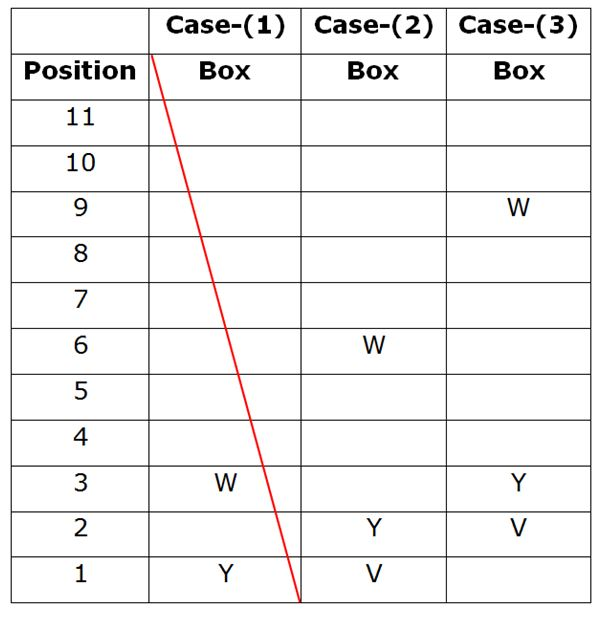• Box X is kept at one of the prime numbered positions.
• Only two boxes are kept between Box S and Box X.
• Box X is placed at one of the positions above Box S.

From the above statements, the prime numbered positions in the above arrangements were- 2, 3, 5, 7 and 11. So, we get,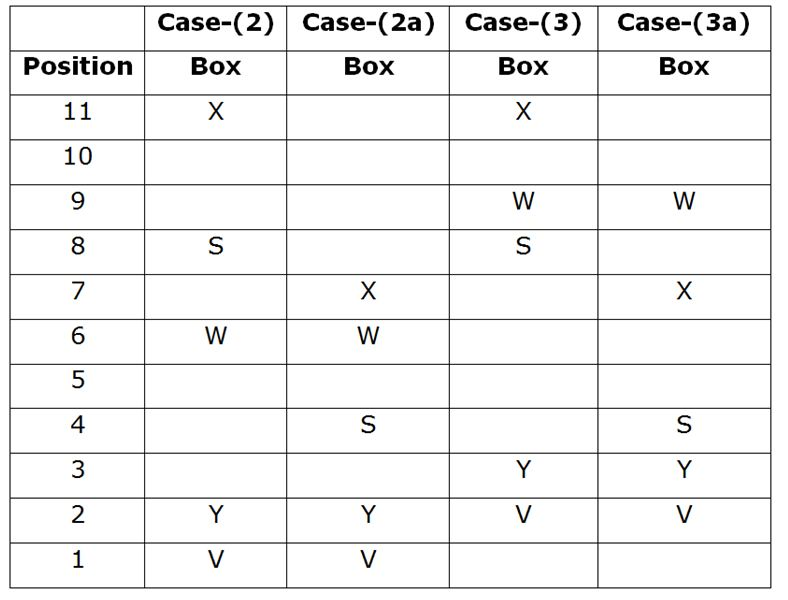• Box P is placed at one of the positions above 6.
• There are two boxes between Box Q and Box P.
• Box P is kept at one of the positions above Box Q.
• Sum of positions of Boxes U and V was equal to the position of Box P.

Since, U+V=P; Case-(2), Case-(2a-i), Case-(3) and Case-(3a) gets eliminated.

From the above statements we get,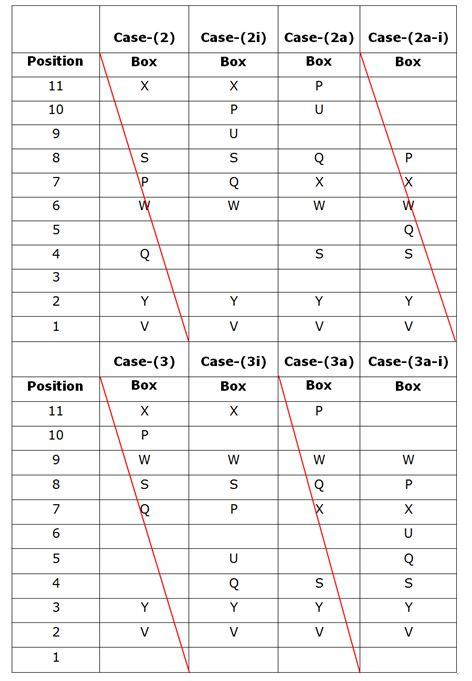• Box Z is kept just above Box T.

So Case-(2a) and Case-(3i) get eliminated.

• At least there are four boxes below Box T.

So Case-(2i) gets eliminated.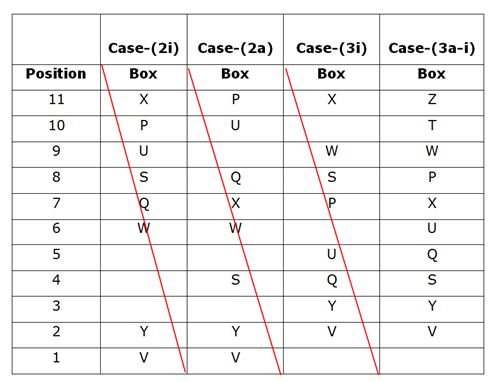So the final arrangement is,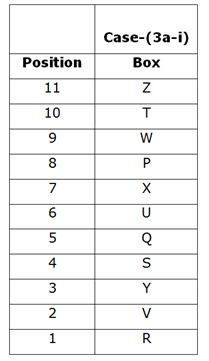Directions (6-9):

456 547 623 526 375

668 668 844 648 486

668  668 448  468  468

456 547 623 526 375

654 745 326 625 573

659 741 331 621 569

1*6=6

456 547 623 526 375

645 466 545 445 564

456 547 623 526 375

654 745  326  625  573

844 935  516  815  763

9+5=14

Given word = “COMPENDIUM”.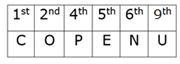Clearly, ‘POUNCE’ can be formed from given letters.

Thus, C is second last letter of the newly formed word.